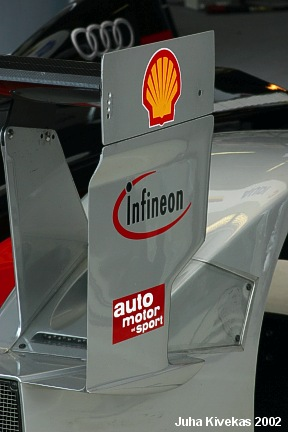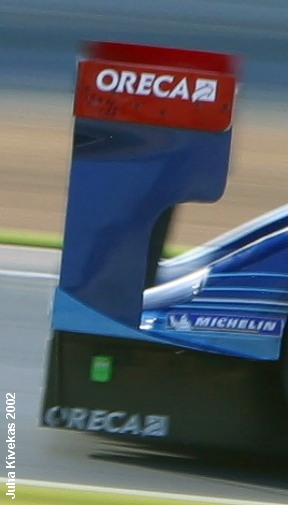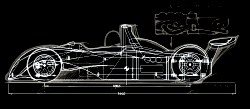Wing Endplate Depth

Wing endplates act as span increase aerodynamically.  That is, in general terms, a wing with an endplate is equal to a wider wing with out one.

>>Using this formula:

Aspect Ratio (AR) = span/chord

We can calculate the AR for wings of 165 cm and 200 cm widths given a chord of 40 cm for both:

165 cm wing AR = 4.125
200 cm wing AR = 5

>>Next, using this formula:

Effective Aspect Ratio (AR_effective) = Actual Aspect Ratio (AR_actual) * (1 + 1.9 * (endplate depth/span))

We can calculate the AR_effective for wings of 165 cm and 200 cm widths given a chord of 40 cm for both, and endplate depths of 15 cm and 60 cm:

165 cm wide wing:
15 cm deep endplate, AR_effective = 4.837
60 cm deep endplate, AR_effective = 6.97

200 cm wide wing:
15 cm deep endplate, AR_effective = 5.7125
60 cm deep endplate, AR_effective = 7.85

>>And finally, going back the original Aspect Ratio formula and using Algebra to work backwards we can then determine the Effective Span, that is, the endplate's impact on aerodynamic span:

The Effective span of a 165 cm wide wing given the endplate depth as such:
15 cm deep endplate = 193.5 cm
60 cm deep endplate = 278 cm

The Effective Span of a 200 cm wide wing given the endplate depth as such:
15 cm deep endplates = 228 cm
60 cm deep endplates = 314 cm

Formulas out of Joseph Katz's New Directions in Race Car Aerodynamics